Courses

# Test: Fourier Series, Numerical Methods & Complex Variables- 2

## 30 Questions MCQ Test Topicwise Question Bank for Mechanical Engineering | Test: Fourier Series, Numerical Methods & Complex Variables- 2

Description
This mock test of Test: Fourier Series, Numerical Methods & Complex Variables- 2 for Civil Engineering (CE) helps you for every Civil Engineering (CE) entrance exam. This contains 30 Multiple Choice Questions for Civil Engineering (CE) Test: Fourier Series, Numerical Methods & Complex Variables- 2 (mcq) to study with solutions a complete question bank. The solved questions answers in this Test: Fourier Series, Numerical Methods & Complex Variables- 2 quiz give you a good mix of easy questions and tough questions. Civil Engineering (CE) students definitely take this Test: Fourier Series, Numerical Methods & Complex Variables- 2 exercise for a better result in the exam. You can find other Test: Fourier Series, Numerical Methods & Complex Variables- 2 extra questions, long questions & short questions for Civil Engineering (CE) on EduRev as well by searching above.
QUESTION: 1

Solution:
QUESTION: 2

### The trigonometric Fourier series for the waveform f(t) shown below contains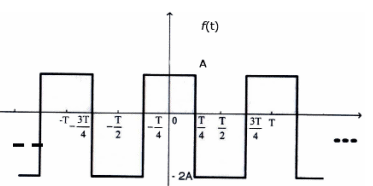Solution:

From figure it’s an even function. so only cosine terms are present in the series and for DC  value,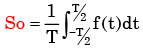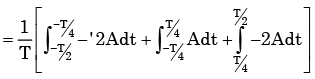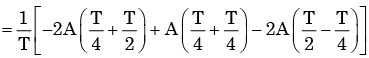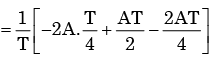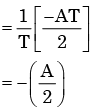So DC take negative value.

QUESTION: 3

### Which of the following functions would have only odd powers of x in its Taylor series expansion about the point x = 0?

Solution: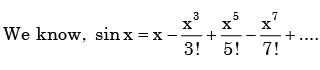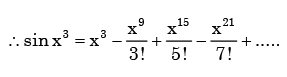QUESTION: 4

The Taylor series expansion of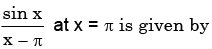Solution:

We know.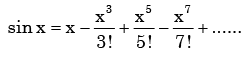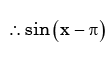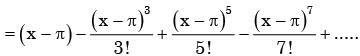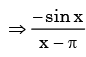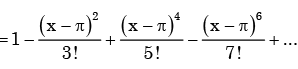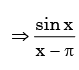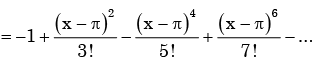QUESTION: 5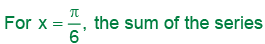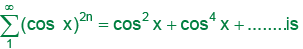Solution: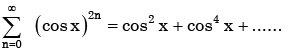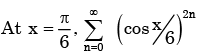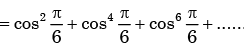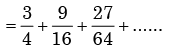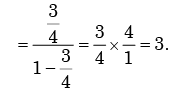QUESTION: 6

The sum of the infinite series,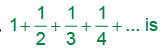Solution: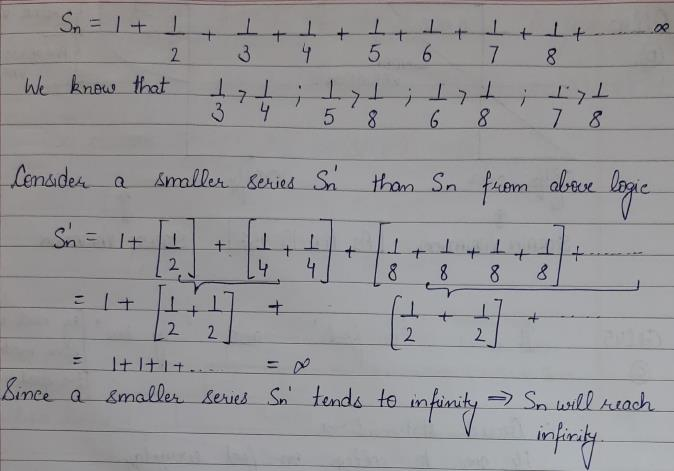QUESTION: 7

The summation of series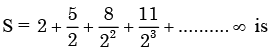Solution: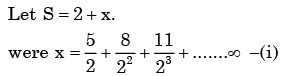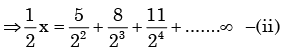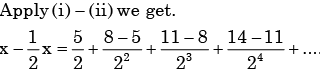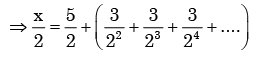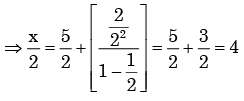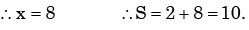QUESTION: 8

Fourier series for the waveform, f (t) shown in fig. is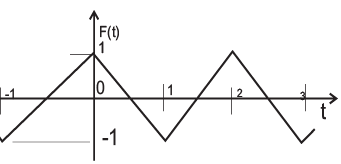Solution:

From the figure, we say f (x) is even functions. so choice (c) is correct.

QUESTION: 9

The Fourier Series coefficients, of a periodic signal x (t), expressed as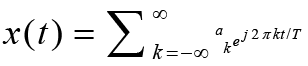are given by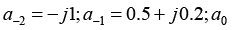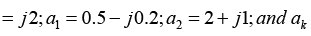Solution:
QUESTION: 10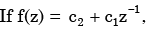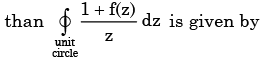Solution: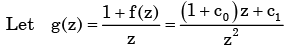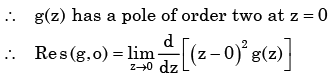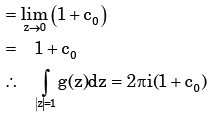QUESTION: 11

For the function of a complex variable W = ln Z (where, W = u + jv and Z = x + jy), the u = constant lines get mapped in Z-plane as

Solution:

Given,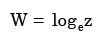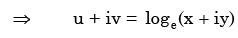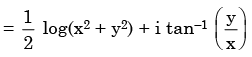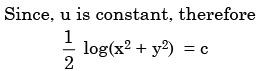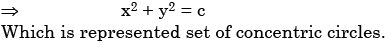QUESTION: 12

ii, where i = √−1, is given by

Solution: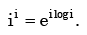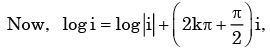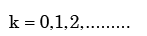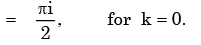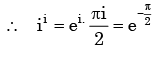QUESTION: 13

Assuming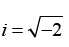and t is a real number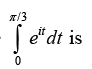Solution: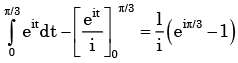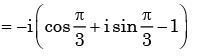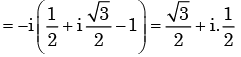QUESTION: 14

The modulus of the complex number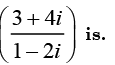Solution: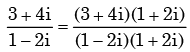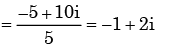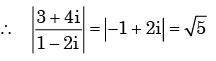QUESTION: 15

Using Cauchy’s integral theorem, the value of the integral (integration being taken in counter clockwise direction)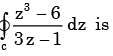Solution: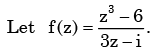Here f (z) has a singularities at z i / 3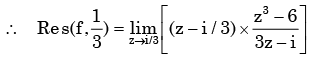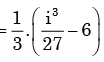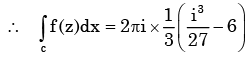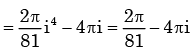QUESTION: 16

Which one of the following is NOT true for complex number Z1and Z2

Solution:

(a) is true since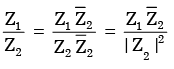(b) is true by triangle inequality of complex number.

(c) is not true since  |Z1 − Z2 |≥|Z1 |– |Z2

(d) is true since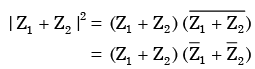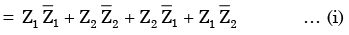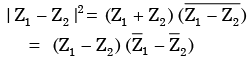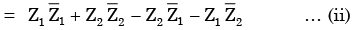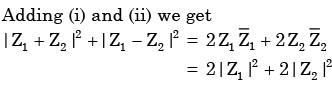QUESTION: 17

For the equation, s3 - 4s2 + s + 6 =0

The number of roots in the left half of s-plane will be

Solution:

Constructing Routh-array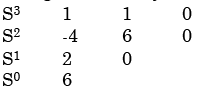Number of sign changes in the first column is two, therefore the number of roots in the left half splane is 2

QUESTION: 18

The value of the integral of the complex function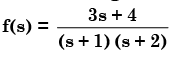Along the path |s| = 3 is

Solution: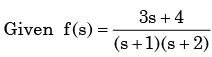f (s) has singularities at s =−1, −2 which are inside the given circle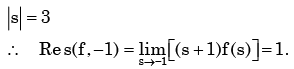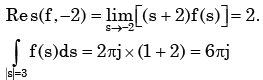QUESTION: 19

For the function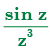of a complex variable z, the point z = 0 is

Solution: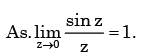Therefore the function has z = 0 is a pole of order 2.

QUESTION: 20

The polynomial p(x) = x5 + x + 2 has

Solution: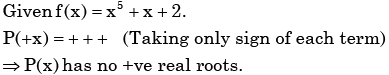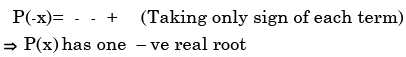As,  P(x) of degree 5  .So other four roots are complex.

QUESTION: 21

If z = x + jy, where x and y are real, the value of |ejz| is

Solution: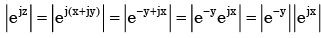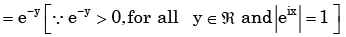QUESTION: 22

The root mean squared value of x(t) = 3 + 2 sin (t) cos (2t) is

Solution:

x(t) = 3 + 2 sin t cos 2t

x(t) = 3 + sin 3t – sin t

∴ Root mean square value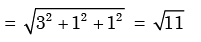QUESTION: 23

We wish to solve x2 – 2 = 0 by Netwon Raphson technique. Let the initial guess b x0 = 1.0 Subsequent estimate of x(i.e.x1) will be:

Solution: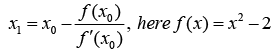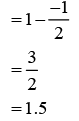QUESTION: 24

The order of error is the Simpson’s rule for numerical integration with a step size h is

Solution:
QUESTION: 25

The table below gives values of a function F(x) obtained for values of x at intervals of 0.25.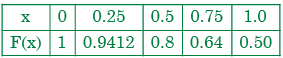The value of the integral of the function between the limits 0 to 1 using Simpson’s rule is

Solution: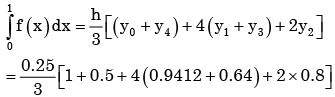QUESTION: 26

A differential equation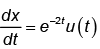has to be solved using trapezoidal rule of integration with a step size h=0.01s. Function u(t) indicates a unit step function. If x(0-)=0, then value of x at t=0.01s will be given by

Solution:
QUESTION: 27

Consider the series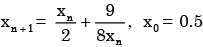obtained from the Newton-Raphson method. The series converges to

Solution: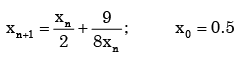The series converges when X n+1= Xn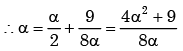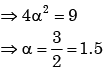QUESTION: 28

Given a>0, we wish to calculate its reciprocal value 1/a by using Newton-Raphson method :
for f(x) = 0. For a=7 and starting wkith x0 = 0.2. the first 2 iterations will be

Solution: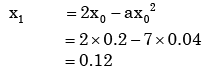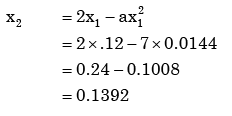QUESTION: 29

With a 1 unit change in b, what is the change in x in the solution of the system of equations x + y = 2, 1.01 x + 0.99 y = b?

Solution:

Given  x + y = 2               …………….. (i)

1.01 x + 0.99 y = b      …………….. (ii)

Multiply 0.99 is equation (i), and subtract from equation (ii), we get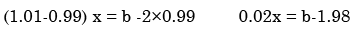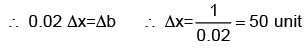QUESTION: 30

The accuracy of Simpson's rule quadrature for a step size h is

Solution: